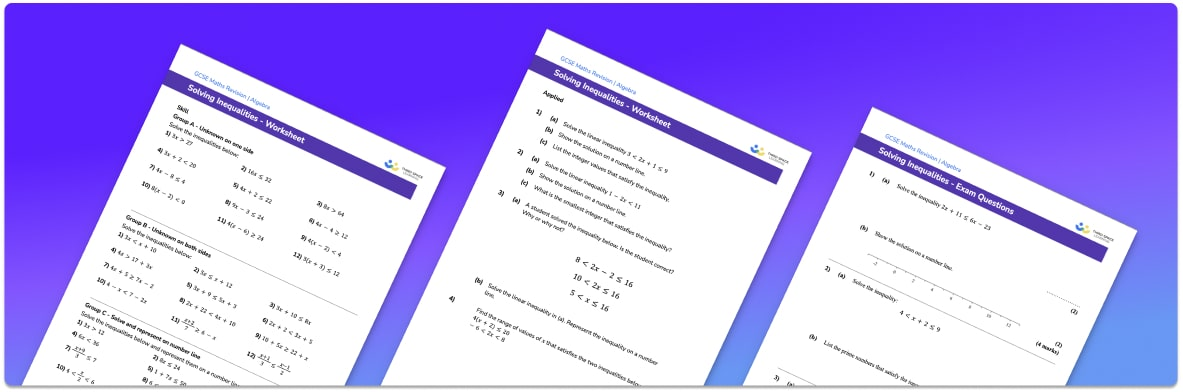# Solving Inequalities Worksheet• Section 1 of the solving inequalities worksheet contains 20+ skills-based solving inequalities questions, in 3 groups to support differentiation
• Section 2 contains 3 applied solving inequalities questions with a mix of worded problems and deeper problem solving questions
• Section 3 contains 3 foundation and higher level GCSE exam questions on solving inequalities
• Answers and a mark scheme for all solving inequalities questions are provided
• Questions follow variation theory with plenty of opportunities for students to work independently at their own level
• All questions created by fully qualified expert secondary maths teachers

Suitable for GCSE maths revision for AQA, OCR and Edexcel exam boards

• This field is for validation purposes and should be left unchanged.

You can unsubscribe at any time (each email we send will contain an easy way to unsubscribe). To find out more about how we use your data, see our privacy policy.

### Solving inequalities at a glance

Inequalities describe the relationship between two expressions. The inequality signs that we use are greater than (>), less than (<), greater than or equal to () and less than or equal to ().

Solving inequalities involves finding the values that make the given inequality true, in the same way that we solve equations. To solve linear inequalities, we use inverse operations to solve the inequality. For example, to solve the two step inequality 3x + 1 < 16, we first subtract 1 to give 3x < 15 and then divide by 3 to give x < 5. The solution is x is less than 5. We could represent the possible values for x on a number line.

Linear inequalities might be one-step inequalities, which only require one inverse operation to solve them, or multi-step inequalities, with a number inverse operations required. The solutions to inequalities could be positive or negative numbers and could be integers, decimals or fractions.

Looking forward, students can then progress to additional inequalities worksheets and other algebra worksheets, for example a sequences worksheet, simultaneous equations worksheet or straight line graphs worksheet.For more teaching and learning support on Algebra our GCSE maths lessons provide step by step support for all GCSE maths concepts.

## Do you have KS4 students who need more focused attention to succeed at GCSE?There will be students in your class who require individual attention to help them succeed in their maths GCSEs. In a class of 30, it’s not always easy to provide.

Help your students feel confident with exam-style questions and the strategies they’ll need to answer them correctly with our dedicated GCSE maths revision programme.

Lessons are selected to provide support where each student needs it most, and specially-trained GCSE maths tutors adapt the pitch and pace of each lesson. This ensures a personalised revision programme that raises grades and boosts confidence.

Find out more